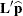ESTIMATE Statement
ESTIMATE ’label’ <fixed-effect values ...>
<| random-effect values ...></ options>
;

The ESTIMATE statement is exactly like a CONTRAST statement, except only one-rowmatrices are permitted. The actual estimate,, is displayed along with its approximate standard error. An approximate t test that= 0 is also produced.

PROC MIXED selects the degrees of freedom to match those displayed in the "Tests of Fixed Effects" table for the final effect you list in the ESTIMATE statement. You can modify the degrees of freedom by using the DF= option.

If PROC MIXED finds the fixed-effects portion of the specified estimate to be nonestimable, then it displays "Non-est" for the estimate entries.

The following examples of ESTIMATE statements compute the mean of the first level of A in the split-plot example (see Example 58.1) for various inference spaces:

```estimate 'A1 mean narrow'   intercept 1
A 1 B .5 .5 A*B .5 .5 |
block   .25 .25 .25 .25
A*Block .25 .25 .25 .25
0   0   0   0
0   0   0   0;
estimate 'A1 mean intermed' intercept 1
A 1 B .5 .5 A*B .5 .5 |
Block .25 .25 .25 .25;
estimate 'A1 mean broad'    intercept 1
A 1 B .5 .5 A*B .5 .5;
```

The construction of thevector for an ESTIMATE statement follows the same rules as listed under the CONTRAST statement.

You can specify the following options in the ESTIMATE statement after a slash (/).

ALPHA=number

requests that a t-type confidence interval be constructed with confidence levelnumber. The value of number must be between 0 and 1; the default is 0.05.

CL

requests that t-type confidence limits be constructed. The confidence level is 0.95 by default; this can be changed with the ALPHA= option.

DF=number

specifies the degrees of freedom for the t test and confidence limits. The default is the denominator degrees of freedom taken from the "Tests of Fixed Effects" table and corresponds to the final effect you list in the ESTIMATE statement.

DIVISOR=number

specifies a value by which to divide all coefficients so that fractional coefficients can be entered as integer numerators.

E

requests that thematrix coefficients be displayed. For ODS purposes, the name of this "L Matrix Coefficients" table is "Coef."

GROUP coeffs
GRP coeffs

sets up random-effect contrasts between different groups when a GROUP= variable appears in the RANDOM statement. By default, ESTIMATE statement coefficients on random effects are distributed equally across groups.

LOWER
LOWERTAILED

requests that the p-value for the t test be based only on values less than the t statistic. A two-tailed test is the default. A lower-tailed confidence limit is also produced if you specify the CL option.

SINGULAR=number

tunes the estimability checking as documented for the SINGULAR= option in the CONTRAST statement.

SUBJECT coeffs
SUB coeffs

sets up random-effect contrasts between different subjects when a SUBJECT= variable appears in the RANDOM statement. By default, ESTIMATE statement coefficients on random effects are distributed equally across subjects. For example, the ESTIMATE statement in the following code from Example 58.5 constructs the difference between the random slopes of the first two batches.

```proc mixed data=rc;
class batch;
model y = month / s;
random int month / type=un sub=batch s;
estimate 'slope b1 - slope b2' | month 1 / subject 1 -1;
run;
```
UPPER
UPPERTAILED

requests that the p-value for the t test be based only on values greater than the t statistic. A two-tailed test is the default. An upper-tailed confidence limit is also produced if you specify the CL option.